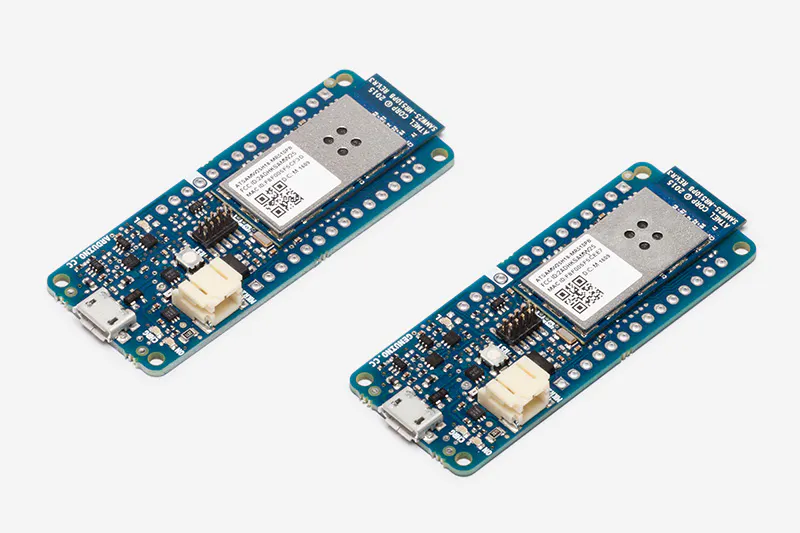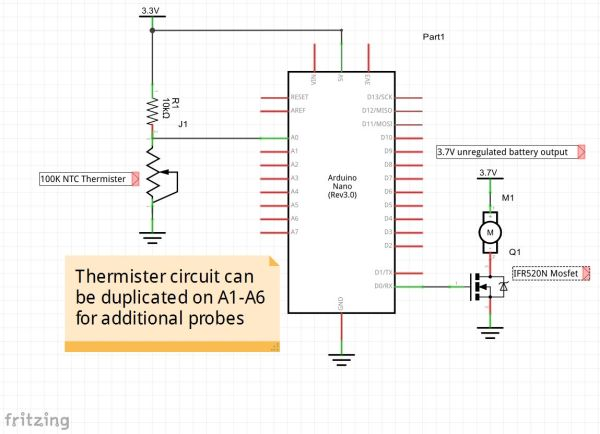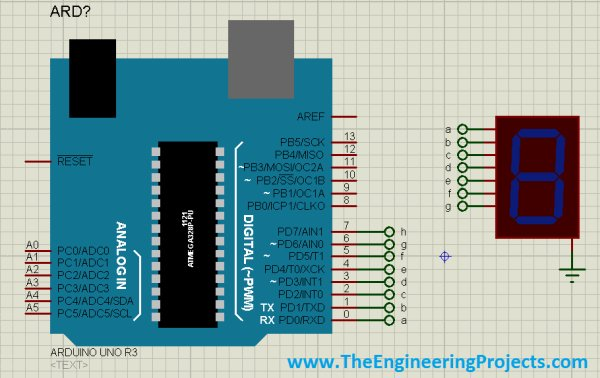# Temperature Controled Charcoal Smoker

Use an Arduino MKR1000 to maintain a constant temperature in a charcoal smoker and allow monitoring over Wifi,## Things used in this project

### Hardware components× 1
 5V Brushless Blower
× 1
 MOSFET IRF520N Driver Module
× 1
 100K ohm NTC Thermistors
× 1× 1Jumper wires (generic)
× 1
 3.7V Lipo Battery >= 700mAh
× 1

### Software apps and online services## Schematics

### Schematic Diagram

The Mrkr1000 is represented by a Arduino Nano
The Fritzing tool did not have the part.## Code

### Smoker Controller Sketch

Arduino

```// sketch_12_04_server_wifi

#include <SPI.h>
#include <WiFi101.h>

char ssid[] = "BeeBar2";  // your network SSID (name)

WiFiServer server(80);
WiFiClient client;

const int numPins = 10;
int pins[] = {, 1, 2, 3, 4, 5, 6, 7, 8, 9};
int pinState[] = {, , , , , , , , , };
char line1;
char buffer;

float targetTemperature = 225;
float smokerTemperature = 225;
float v0;

void setup()
{
Serial.begin(9600);
while (!Serial) {}; // Leonardo needs this
for (int i = ; i < numPins; i++)
{
pinMode(pins[i], OUTPUT);
}
// change the ADC resolution to 12 bits

if (WiFi.begin(ssid, pass))
{
WiFi.config(server_IP);
printWifiStatus();
//Serial.println(WiFi.localIP());
}
else
{
Serial.println("Could not connect to network");
}
server.begin();
}

void loop()
{
client = server.available();
if (client)
{
if (client.connected())
{
Serial.println(line1);

if (! pageNameIs("/"))
{
Serial.print("Invalid Page Requested: ");
Serial.println(line1);
client.stop();
return;
}
client.println("HTTP/1.1 200 OK\nContent-Type: text/html\n");
sendBody();
delay(1);
client.stop();
}
else
{
Serial.println("No Client connected ");
}
}
v0 = (float) reading / 1241.0;
smokerTemperature = ThermisterTemp(v0, 100000, 10000, 3950, 25);
digitalWrite(, smokerTemperature<targetTemperature);
}

void sendBody()
{
client.println("<html><body>");
client.println("<form method='GET'>");
client.print("\n<h1>Target Temperature:</h1> <input type='number' value='");
client.print(targetTemperature);
client.println("' name='t'><br>");
client.println("<input type='submit' value='Update'/>\n</form>\n</body></html>");
}

{
client.println("<h1>Temperatures</h1>\n<table border='1'>");

Serial.print("Pin(");Serial.print();Serial.print("): V=");Serial.print(v0);Serial.print(" Temperature="); Serial.println(smokerTemperature);

client.print("<tr><td>Smoker ");
client.print("</td><td>"); client.print(smokerTemperature);
client.println(" F</td></tr>");

for (int i = 1; i < 7; i++)
{
{
float v = (float) reading / 1241.0;
float T = ThermisterTemp(v, 100000, 10000, 3950, 25);
client.print("<tr><td>Probe "); client.print(i);
client.print("</td><td>"); client.print(T);
client.println(" F</td></tr>");
}
}
client.println("</table>");
}

{
while (readRequestLine(buffer) > 1 && buffer[] != '\n') {}
}

{
char ch;
int i = ;
while (ch != '\n' && i < 100 && client.available())
{
if (client.available())
{
line[i] = ch;
i ++;
}
}
line[i] = '\0';
return i;
}

boolean pageNameIs(char* name)
{
// page name starts at char pos 4
// ends with space
int i = 4;
char ch = line1[i];
while (ch != ' ' && ch != '\n' && ch != '?')
{
if (name[i - 4] != line1[i])
{
return false;
}
i++;
ch = line1[i];
}
return true;
}

{
//Serial.print("parsing targetTemperature");Serial.println(line1);

int len = strlen(line1);
for (int i = ; i < len; i++)
{
if (line1[i] == 't' && line1[i + 1] == '=')
{
float val = ;
for (int j=i+2; j< len; j++)
{
if (isDigit(line1[j]))
{
//Serial.print(line1[j]);
val *= 10;
val += (int)line1[j]-'0';
}
else
{
break;
}
}
//Serial.print(" ");
targetTemperature = val;
//Serial.print("targetTemperature = ");Serial.println(val);
return val;
}
}
return -1;
}

void printWifiStatus() {
// print the SSID of the network you're attached to:
Serial.print("SSID: ");
Serial.println(WiFi.SSID());

Serial.println(ip);

// print the received signal strength:
Serial.println(" dBm");
}

float ThermisterTemp(float Vtherm, int R0therm, int Rfixed, int Beta, int T0)
{
// Current throught the 2 rsiteres is the same, so compute the current
// throught the fixed resiter using Ohms law I = V / R
// The voltage drop over the fixed resister is Vcc (3.3V) - Vtherm
double I = (3.3 - Vtherm) / Rfixed;
//Serial.print("I=");Serial.print(I,12);Serial.print(" ");

// Use ohms law to cacultate the resitance of the thermister R = V / I
double Rtherm = Vtherm / I;
//Serial.print("Rtherm=");Serial.print(Rtherm);Serial.print(" ");

// Use the Beta eaquation to calulate the tempuratue in C
// 1/T = (1/Beta)*ln(Rtherm/R0therm) + (1/T0)
// (Steinhart-Hart equatin is more accutrate, but I only have the Beta constant
// for my thermister.

// All temperatres are in Kelvin
T0 += 273.15;

double T = 1.0/( (1.0/((double)Beta))*log((Rtherm)/((double)R0therm)) + (1.0/((double)T0)) );
//Serial.print("T=");Serial.print(T);Serial.print(" ");

//Serial.println("");
// convert to farenheit for us ignorant Americans
return (float) ((9*(T-273.15))/5)+32;
}
```

#### This Post / Project can also be found using search terms:

• arduino temperature controlled smoker